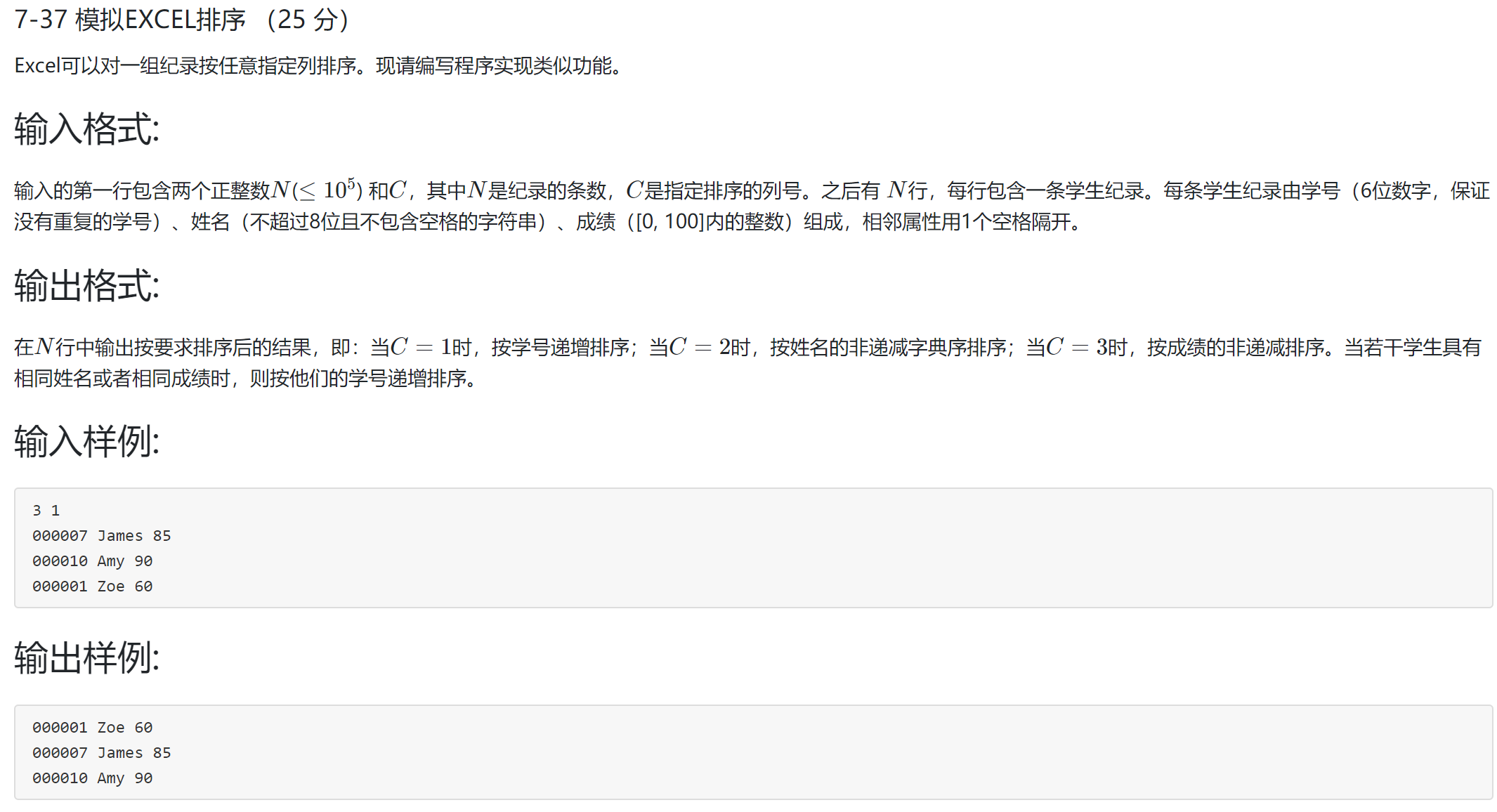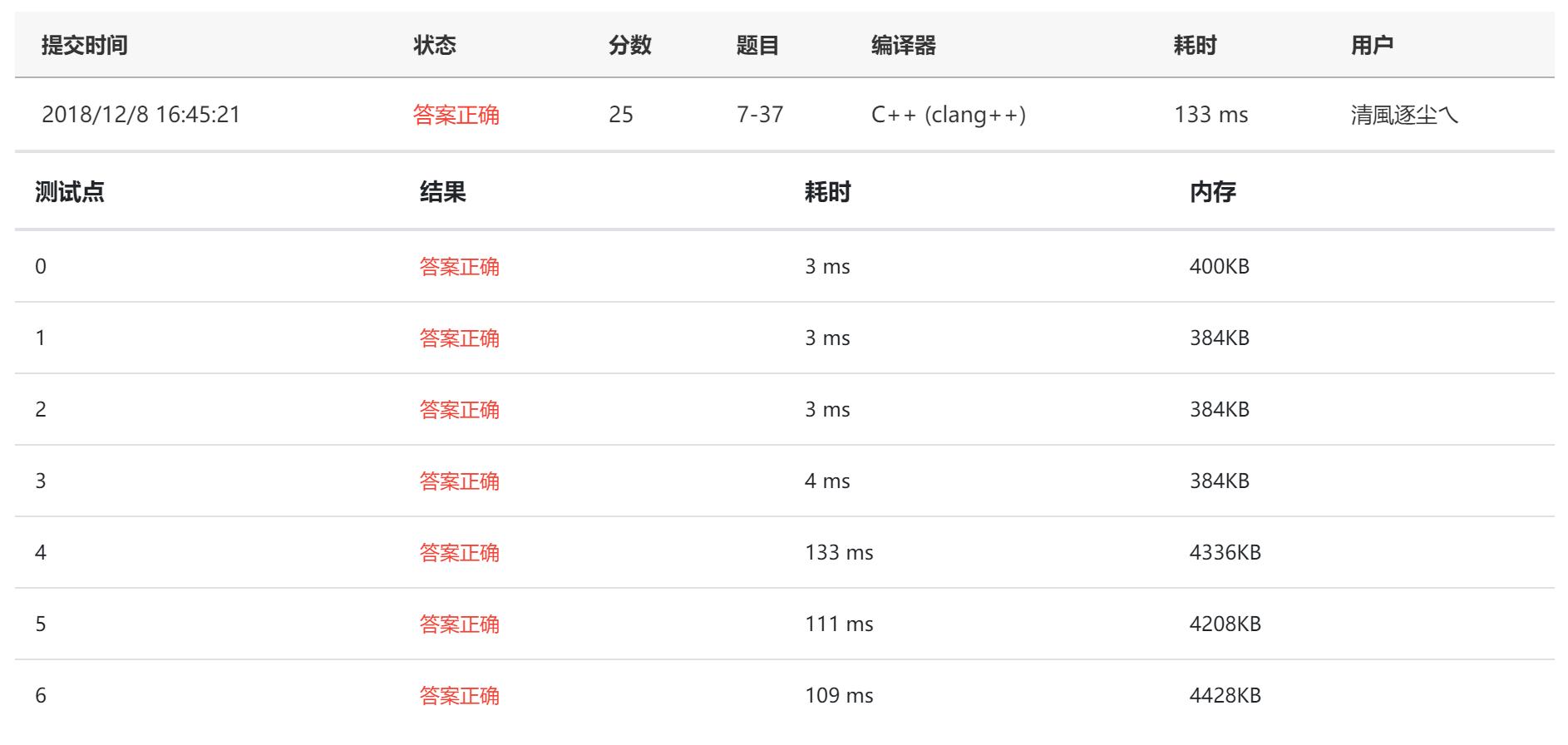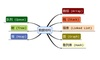• #include<bits/stdc++.h> using namespace std; const int maxn = 1e5 + 10; int n, c; struct student{ ...void heapAjust(int head, int tail, bool (*cmp)(student, student)){ student value.#include<bits/stdc++.h>
using namespace std;

const int maxn = 1e5 + 10;
int n, c;
struct student{
char num;
char name;
}stu[maxn];

void heapAjust(int head, int tail, bool (*cmp)(student, student)){
int id;//较大的孩子
while(l<=tail){
if(r<=tail && (cmp(stu[l], stu[r]))) id = r;
else id = l;
if((cmp(value, stu[id]))){//堆顶小
head = id; l = id*2; r = id*2+1;
}
else break;
}

void heapSort(bool (*cmp)(student, student)){
for(int i = n/2; i > 0; i--)
heapAjust(i, n, cmp);
for(int i = 1; i <= n-1; i++){
student tmp = stu;
stu = stu[n-i+1];
stu[n-i+1] = tmp;
heapAjust(1, n-i, cmp);
}
}
bool cmp1(student a, student b){
if(strcmp(a.num, b.num)<0) return 1;
return 0;
}
bool cmp2(student a, student b){
if(strcmp(a.name, b.name) == 0) return cmp1(a, b);
if(strcmp(a.name, b.name)<0) return 1;
return 0;
}
bool cmp3(student a, student b){
}
int main(){
scanf("%d%d",&n,&c);
for(int i = 1; i <= n; i++)
if(c==1) heapSort(cmp1);//sort(stu+1, stu+n+1, cmp1);
else if(c==2) heapSort(cmp2);//sort(stu+1, stu+n+1, cmp2);
else if(c==3) heapSort(cmp3);//sort(stu+1, stu+n+1, cmp3);
for(int i = 1; i <= n; i++)
return 0;
}

仅供参考 切勿抄袭
hang hang hang !!!

展开全文数据结构
• 我的数据结构与算法题目集代码仓：https://github.com/617076674/Data-structure-and-algorithm-topic-set 原题链接：https://pintia.cn/problem-sets/15/problems/864 题目描述： 知识点：排序 思路：用sort...

题目描述：知识点：排序

思路：用sort函数排序

时间复杂度是O(NlogN)。空间复杂度是O(N)。

C++代码：

#include<iostream>
#include<algorithm>
#include<cstring>
#include<vector>

using namespace std;

struct student{
int number;
char name;
int score;
};

int N, C;
vector<student> students;

bool cmp(student s1, student s2);

int main(){
scanf("%d %d", &N, &C);
for(int i = 0; i < N; i++){
int number;
char name;
int score;
scanf("%d %s %d", &number, name, &score);
student stu;
stu.number = number;
strcpy(stu.name, name);
stu.score = score;
students.push_back(stu);
}
sort(students.begin(), students.end(), cmp);
for(int i = 0; i < N; i++){
printf("%06d %s %d\n", students[i].number, students[i].name, students[i].score);
}
return 0;
}

bool cmp(student s1, student s2){
if(C == 1){
return s1.number < s2.number;
}else if(C == 2){
int cmpName = strcmp(s1.name, s2.name);
if(cmpName == 0){
return s1.number < s2.number;
}else{
return cmpName < 0;
}
}else{
if(s1.score == s2.score){
return s1.number < s2.number;
}else{
return s1.score < s2.score;
}
}
}

C++解题报告：展开全文数据结构与算法题目集 排序 模拟EXCEL排序
• 写这个是因为自己在学习数据结构的时候去网上找的答案大部分都是C++的 但是没有C的 这让用C写的我非常崩溃 所以在写完之后想要把自己的C代码和大家分享一下 下面进入正题 7-37 Excel可以对一组纪录按任意指定列排序...

第一次写文章 不喜勿喷~
写这个是因为自己在学习数据结构的时候去网上找的答案大部分都是C++的 但是没有C的 这让用C写的我非常崩溃 所以在写完之后想要把自己的C代码和大家分享一下
下面进入正题

7-37 Excel可以对一组纪录按任意指定列排序。现请编写程序实现类似功能。

输入格式:

输入的第一行包含两个正整数N(≤10
​5
​​ ) 和C，其中N是纪录的条数，C是指定排序的列号。之后有 N行，每行包含一条学生纪录。每条学生纪录由学号（6位数字，保证没有重复的学号）、姓名（不超过8位且不包含空格的字符串）、成绩（[0, 100]内的整数）组成，相邻属性用1个空格隔开。

输出格式:

在N行中输出按要求排序后的结果，即：当C=1时，按学号递增排序；当C=2时，按姓名的非递减字典序排序；当C=3时，按成绩的非递减排序。当若干学生具有相同姓名或者相同成绩时，则按他们的学号递增排序。

输入样例:

3 1
000007 James 85
000010 Amy 90
000001 Zoe 60
输出样例:

000001 Zoe 60
000007 James 85
000010 Amy 90

这道题就是使用快速排序就可以了 但是需要注意一点的是 这里要使用三数取中法来对付N最大的C=1，C=2 的情况 ，不知道是不是给出的测试数据的顺序对和逆序对个数相近 我在用非三数取中的快速排序的测试情况下给出了TLE 但是C=3 的却是AC
下面放失败的图以及代码

#include<stdio.h>
#include<string.h>
#include<malloc.h>

struct Student{
char name;
int ID;
int score;
};

int cmp(struct Student *s1,struct Student *s2,int way);
void QuickSort(struct Student *student,int left,int right,int way);

int main(void)
{
int N,C;    //记录条数  排序方式
int i;
fscanf(stdin,"%d %d",&N,&C);
struct Student *student;
student=(struct Student *)malloc(N*sizeof(struct Student));

for(i=0;i<N;i++)
fscanf(stdin,"%d %s %d",&student[i].ID,student[i].name,&student[i].score);

QuickSort(student,0,N-1,C);

for(i=0;i<N;i++)
printf("%06d %s %d\n",student[i].ID,student[i].name,student[i].score);

free(student);

return 0;
}

int cmp(struct Student *s1,struct Student *s2,int way)
{
int result=0;

if(1==way)
result=s2->ID-s1->ID;
else if(2==way){
result=strcmp(s1->name,s2->name);
result*=-1;
}
else
result=s2->score-s1->score;

if(!result)
result=s2->ID-s1->ID;

return result;
}

void QuickSort(struct Student *student,int left,int right,int way)
{
if(left>=right)
return ;

int i,j;
struct Student temp;
i=left;
j=right;

while(i<j){
while(i<j&&cmp(&student[left],&student[j],way)>0)
j--;
while(i<j&&cmp(&student[left],&student[i],way)<=0)
i++;
if(i<j){
temp=student[i];
student[i]=student[j];
student[j]=temp;
}
}

if(left!=i){
temp=student[left];
student[left]=student[i];
student[i]=temp;
}

QuickSort(student,left,i-1,way);
QuickSort(student,i+1,right,way);

return ;
}

然后在翻阅别人的代码是发现用得是C++中的 qsort 函数 去查阅代码的时候发现它是先进行1.5*log2(n)次的快排，如果还没排完，就使用堆排
这两种排序的复杂度相等，然后我就想到了堆栈溢出的问题 ，当基本有序的时候快排会造成堆栈的大量堆积，这样可能造成堆栈溢出 。然后一看数据量是10^5 量级 然后用该量级的完全有序数据去跑了下 给了段错误 于是判断就是堆栈溢出的错误 采用三数取中后就AC掉了

#include<stdio.h>
#include<string.h>
#include<malloc.h>

struct Student{
char name;
int ID;
int score;
};

int cmp(struct Student *s1,struct Student *s2,int way);
void QuickSort(struct Student *student,int left,int right,int way);

int main(void)
{
int N,C;    //记录条数  排序方式
int i;
fscanf(stdin,"%d %d",&N,&C);
struct Student *student;
student=(struct Student *)malloc(N*sizeof(struct Student));

for(i=0;i<N;i++)
fscanf(stdin,"%d %s %d",&student[i].ID,student[i].name,&student[i].score);

QuickSort(student,0,N-1,C);

for(i=0;i<N;i++)
printf("%06d %s %d\n",student[i].ID,student[i].name,student[i].score);

free(student);

return 0;
}

int cmp(struct Student *s1,struct Student *s2,int way)
{
int result=0;

if(1==way)
result=s2->ID-s1->ID;
else if(2==way)
result=strcmp(s2->name,s1->name);
else
result=s2->score-s1->score;

if(!result)
result=s2->ID-s1->ID;

return result;
}

void QuickSort(struct Student *student,int left,int right,int way)
{
if(left>=right)
return ;

int i,j,mid;
int index;

struct Student temp;
i=left;
j=right;
mid=left+((right-left)>>1);

if(3==way)
index=left;              // 按成绩排序的时候 因为最多只有100次的分层 故可以不用三数取中 这样节省了比较的时间
else{
if(cmp(&student[left],&student[right],way)>0){
if(cmp(&student[left],&student[mid],way)>0){
if(cmp(&student[mid],&student[right],way)>0)
index=mid;
else
index=right;
}
else
index=left;
}
else{
if(cmp(&student[mid],&student[right],way)>0)
index=right;
else{
if(cmp(&student[mid],&student[left],way)>0)
index=mid;
else
index=left;
}
}                            //三数取中法
}

while(i<j){
while(i<j&&cmp(&student[index],&student[j],way)>0)
j--;
while(i<j&&cmp(&student[index],&student[i],way)<=0)
i++;
if(i<j){
temp=student[i];
student[i]=student[j];
student[j]=temp;
}
}

if(index!=i){
temp=student[index];
student[index]=student[i];
student[i]=temp;
}

QuickSort(student,left,i-1,way);
QuickSort(student,i+1,right,way);

return ;
}展开全文C语言
• 7-19 模拟EXCEL排序 (25分) Excel可以对一组纪录按任意指定列排序。现请编写程序实现类似功能。 输入格式: 输入的第一行包含两个正整数N(≤10e​5​​ ) 和C，其中N是纪录的条数，C是指定排序的列号。之后有 N行，每...

7-19 模拟EXCEL排序 (25分)

Excel可以对一组纪录按任意指定列排序。现请编写程序实现类似功能。

输入格式:

输入的第一行包含两个正整数N(≤10e​5​​ ) 和C，其中N是纪录的条数，C是指定排序的列号。之后有 N行，每行包含一条学生纪录。每条学生纪录由学号（6位数字，保证没有重复的学号）、姓名（不超过8位且不包含空格的字符串）、成绩（[0, 100]内的整数）组成，相邻属性用1个空格隔开。

输出格式:

在N行中输出按要求排序后的结果，即：当C=1时，按学号递增排序；当C=2时，按姓名的非递减字典序排序；当C=3时，按成绩的非递减排序。当若干学生具有相同姓名或者相同成绩时，则按他们的学号递增排序。

输入样例:

3 1
000007 James 85
000010 Amy 90
000001 Zoe 60

输出样例:

000001 Zoe 60
000007 James 85
000010 Amy 90

Acceped Code

#include <iostream>
#include <algorithm>
#include <iomanip>
using namespace std;
struct Node {
int Num;
string name;
int Score;
};
bool cmp1 (Node a, Node b) {
return a.Num < b.Num;
};
bool cmp2 (Node a, Node b) {
if (a.name == b.name)
return a.Num < b.Num;
return a.name < b.name;
};
bool cmp3 (Node a, Node b) {
if (a.Score == b.Score)
return a.Num < b.Num;
return a.Score < b.Score;
};

Node List;
int main() {
int N, C;
scanf("%d%d", &N, &C);
for (int i = 0; i < N; ++i) {
cin >> List[i].Num >> List[i].name >> List[i].Score;
}
if (C == 1) {
sort(List, List + N, cmp1);
}
if (C == 2) {
sort(List, List + N, cmp2);
}
if (C == 3) {
sort(List, List + N, cmp3);
}
for (int i = 0; i < N; ++i) {
cout << setw(6) << setfill('0') << List[i].Num << " " << List[i].name
<< " " << List[i].Score << endl;
}
return 0;
}

展开全文• Excel可以对一组纪录按任意指定列排序。现请编写程序实现类似功能。 输入格式: 输入的第一行包含两个正整数N(≤10​^5) 和C，其中N是纪录的条数，C是指定排序的列号。之后有 N行，每行包含一条学生纪录。每条学生...字符串 排序算法 数据结构
• 模拟Excel排序

2014-12-31 17:16:38
一个简单的模拟Excel排序代码，可以实现Excel的排序功能，可以根据排序方式进行排序数据结构
• Excel可以对一组纪录按任意指定列排序。现请编写程序实现类似功能。 输入格式: 输入的第一行包含两个正整数N(≤10 ​5 ​​ ) 和C，其中N是纪录的条数，C是指定排序的列号。之后有 N行，每行包含一条学生纪录。每条...c语言 数据结构 算法
• Excel可以对一组纪录按任意指定列排序。现请编写程序实现类似功能。 输入格式: 输入的第一行包含两个正整数N(≤10 ​5 ​​ ) 和C，其中N是纪录的条数，C是指定排序的列号。之后有 N行，每行包含一条学生纪录。每条...
• 原题链接 怎么说呢，这道题我偷懒了。 我必须要承认，我偷懒了。 但是还是必须要说stdlib.h库中的qsort函数是真的牛逼。 建议大家先百度这个函数再来看我的代码，这样会很好懂。 #include <stdio.h>...算法 c语言 数据结构
• 7-37 模拟EXCEL排序 （25 分)Excel可以对一组纪录按任意指定列排序。现请编写程序实现类似功能。 输入格式: 输入的第一行包含两个正整数N(≤10​5​​) 和C，其中N是纪录的条数，C是指定排序的列号。之后有 N行，每...
• 用vector配合string进行存储，函数指针pfun根据flag值指向对应的比较函数，排序后输出。 #include<iostream> #include<vector> #include<algorithm> #include<string> using namespace ...c++ 算法 数据结构
• 5-3 模拟EXCEL排序 (25分)

千次阅读 2017-06-04 16:17:25
Excel可以对一组纪录按任意指定列排序。现请编写程序实现类似功能。 输入格式: 输入的第一行包含两个正整数NN(\le 10^5≤10​5​​) 和CC，其中NN是纪录的条数，CC是指定排序的列号。之后有 NN行，每行...c语言 数据结构
• 7-37模拟EXCEL排序(25分) Excel可以对一组纪录按任意指定列排序。现请编写程序实现类似功能。 输入格式: 输入的第一行包含两个正整数N(≤10​5​​) 和C，其中N是纪录的条数，C是指定排序的列号。之后有N行，每行...
• 7-22 模拟EXCEL排序 (25分) Excel可以对一组纪录按任意指定列排序。现请编写程序实现类似功能。 输入格式: 输入的第一行包含两个正整数N(≤10^5 ​​ ) 和C，其中N是纪录的条数，C是指定排序的列号。之后有 N行，每...数据结构 字符串 排序算法 过拟合
• 5-37 模拟EXCEL排序 (25分)

千次阅读 2016-08-30 11:39:06
5-37 模拟EXCEL排序 (25分)Excel可以对一组纪录按任意指定列排序。现请编写程序实现类似功能。 输入格式:输入的第一行包含两个正整数N(105 10^5​​) 和C，其中N是纪录的条数，C是指定排序的列号。之后有 N行，每行...c语言 排序
• 7-37 模拟EXCEL排序 (25 point(s)) Excel可以对一组纪录按任意指定列排序。现请编写程序实现类似功能。 输入格式: 输入的第一行包含两个正整数N(≤105) 和C，其中N是纪录的条数，C是指定排序的列号。之后有 N行，每...
• Excel可以对一组纪录按任意指定列排序。现请编写程序实现类似功能。 输入格式: 输入的第一行包含两个正整数N(≤10​5​​) 和C，其中N是纪录的条数，C是指定排序的列号。之后有N行，每行包含一条学生纪录。每条...
• Excel可以对一组纪录按任意指定列排序。现请编写程序实现类似功能。 输入格式: 输入的第一行包含两个正整数N(≤10​5​​) 和C，其中N是纪录的条数，C是指定排序的列号。之后有 N行，每行包含一条学生纪录。每条...
• 7-1 模拟EXCEL排序 (25 分) Excel可以对一组纪录按任意指定列排序。现请编写程序实现类似功能。 输入格式: 输入的第一行包含两个正整数N(≤10 ​5 ​​ ) 和C，其中N是纪录的条数，C是指定排序的列号。之后有 N行，...
• 数据结构】—模拟实现顺序表(C语言) 引言：顺序表是数据结构的一种简单线性结构，在学习过程中，刚从C语言过渡到数据结构，承前启后，既考验C语言语法功底，又考验了对数据结构入门知识的了解。 定义：顺序表是用...
• HDU1862EXCEL排序

2014-03-26 22:12:51
其实最近都没有兴趣做排序题目，因为我觉得纯粹排序对我而言进步不大，但是舍友TLE了，叫我试一试。 整道题的思路很简单啦，我用的是快排，比较的原则也给得很清楚，不必多言，我没有用stdlib的快排，也没有用scanf...
• EXCEL排序

2020-05-19 21:52:22
Excel可以对一组纪录按任意指定列排序。现请你编写程序实现类似功能。 对每个测试用例，首先输出1行“Case i:”，其中 i 是测试用例的编号（从1开始）。随后在 N 行中输出按要求排序后的结果，即：当 C=1 时，按学号...c++ 数据结构
• R7-9 模拟EXCEL排序 (25 分) Excel可以对一组纪录按任意指定列排序。现请编写程序实现类似功能。 输入格式: 输入的第一行包含两个正整数N(≤105) 和C，其中N是纪录的条数，C是指定排序的列号。之后有N行，每行...c++ 数据结构
• Excel可以对一组纪录按任意指定列排序。现请编写程序实现类似功能。 输入格式: 输入的第一行包含两个正整数N(≤10^5) 和C，其中N是纪录的条数，C是指定排序的列号。之后有 N行，每行包含一条学生纪录。每条学生纪录...数据结构 排序算法 c++...

数据结构模拟excel排序数据结构 订阅